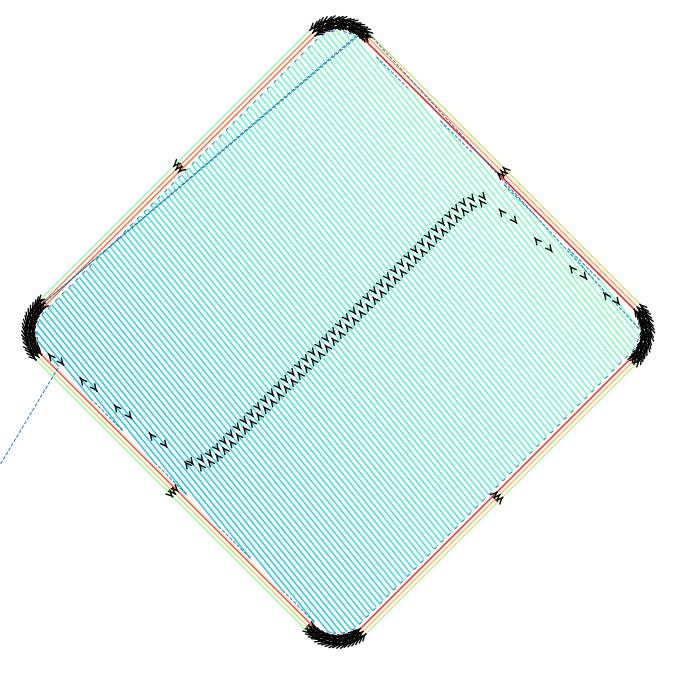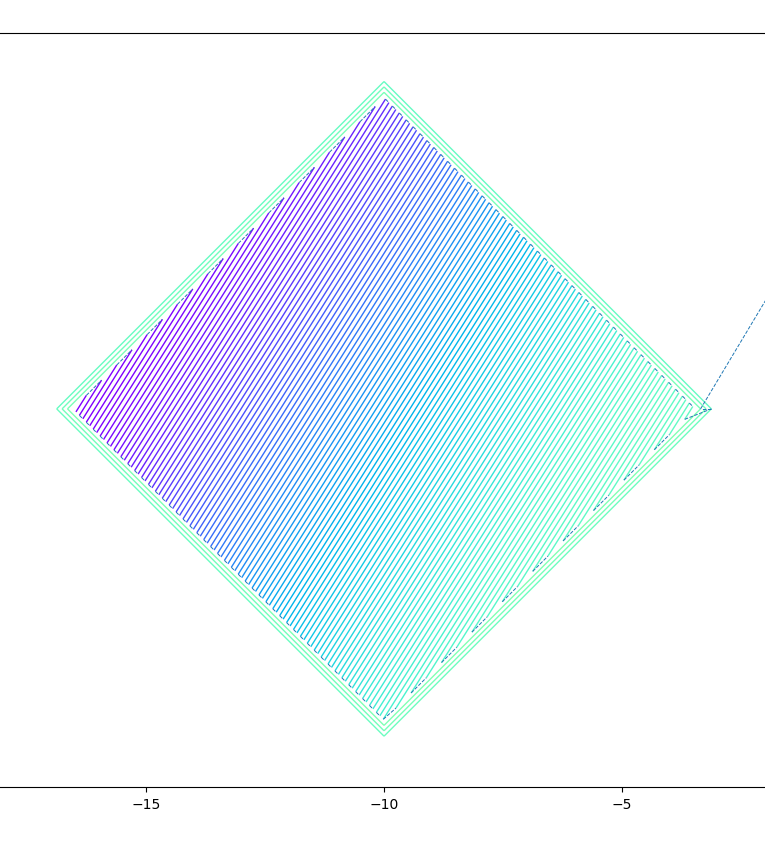Tag: Visualisation

# PySLM: Layer Visualisation Update

Following a recent suggestion, it was useful to revisit the basic visualisation functionality within PySLM for visualising LayerGeometry in a Layer. The request suggested was related to displaying jump vectors between vectors and implementing this within the visualisation. Jump vectors visualise, the jump across adjacent scan vectors when the laser or energy source is not firing . Although it is a trivial matter, good visualisation helps us to understand how scan vectors are processed by the additive manufacturing machine.

The current implementation does visualise the order of scanning but not correctly in its previous form. All HatchGeometry and ContourGeometry is gathered across the layer and processed separately. The order of scanning within the HatchGeometry is found by collecting across all the scan vectors and simply assigning a range across all scan vectors, using numpy.arange.

# Plot the sequential index of the hatch vector
lc.set_array(np.arange(len(hatches)))

Obviously, the current approach isolates the hatch and contour scan vectors so even when the contour vectors are scanned first or there is an arbitrary order of scanning across types – especially for complex scan strategy arrangements, the visualisation is incorrect. Likewise, for displaying the jump vectors, this would not work correctly.

### New Approach

Restarting, the approach simply takes existing collection of LayerGeometry and then gathers together all the vectors across both HatchGeometry and ContourGeometry object. However, ContourGeometry vectors are joined together by coordinates rather than discrete pair of coordinates representing the vectors. Across the ContourGeometry, the vectors are transformed into the equivalent hatch vectors in order to be consistent when using Matplotlib’s LineCollection objects. This is done by stacking the ContourGeometry’s coordinates by offsetting by a single coordinate position.

scanVectors = []
for geom in layer.geometry:

if isinstance(geom, HatchGeometry):
coords = geom.coords.reshape(-1, 2, 2)
elif isinstance(geom, ContourGeometry):
coords = np.hstack([geom.coords, np.roll(geom.coords, -1, axis=0)]).reshape(-1,2,2)

scanVectors.append(coords)

scanVectors = np.vstack(scanVectors)

These can then be plotted using Matplotlib LineCollection:

lc = matplotlib.collections.LineCollection(scanVectors, cmap=plt.cm.rainbow, linewidths=1.0)

Unfortunately, there is some inefficiency merging all the scan vectors due to copy operations and stacking the array structure, although for the purpose of visualising single layers it is satisfactory.

Depending on the geometry, especially those with many facets in the mesh, the slicing results in contours which contain many edges, that may persist even after polygonal simplification. For representing the scan order by changing the colour hue, this may result in colour map biased with the contours when attempting to visualise the order.The range of colour are limited across all the scan vectors due to the large number of edges on the outer border

A method to circumvent this is to use the cumulative distance across each scan vector that is taken across the entire layer. This acts as a way to normalise the scan order irrespective of complexity, length and distribution of scan vectors across the entire length.

delta = scanVectors[:, 1, :] - scanVectors[:, 0, :]
dist = np.sqrt(delta[:, 0] * delta[:, 0] + delta[:, 1] * delta[:, 1])
cumDist = np.cumsum(dist)
lc.set_array(cumDist.ravel())

The final step is accounting for the jump vectors i.e. when the galvo mirrors move between scan vectors without the energy source activated. Having a consistent list of scan vectors means this is straightforward to calculate by replicating the same technique used for translating the ContourGeometry into hatches:

scanVectors = np.vstack(scanVectors)
svTmp = scanVectors.copy().reshape(-1,2)
svTmp = np.roll(svTmp,-1,axis=0)[0:-2]
svTmp = svTmp.reshape(-1,2,2)

lc2 = mc.LineCollection(svTmp, cmap=plt.cm.get_cmap('Greys'), linewidths=0.3, linestyles="--", lw=0.7)

This involves taking every odd pair across all the scan vector lists. These are plotted as line collections with dashes – that are more appealing on the eye. However, this results in ‘invisible’ jump lines between the scan vectors during Contour scans. Nevertheless, it presents a simple approach to generating more informative visuals for the scan vector paths across the layer. The final result is shown below.New sequential visualisation showing sequential scanning of both hatch and contour geometries with jump vectors (dashed lines)

This new function for plotting can be found in pyslm.visualise.plotSequential.